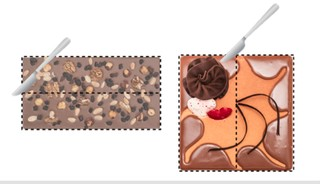Dividing a figure into equal parts

# Dividing a figure into equal parts

Students learn to divide a figure into equal parts.

No account needed.8,000 schools use Gynzy92,000 teachers use Gynzy1,600,000 students use Gynzy

## General

The students learn to divide a figure into equal parts.

## Standards

CCSS.Math.Content.2.G.A.3

## Learning objective

Students will be able to divide a figure into equal parts.

## Introduction

Have the students determine whether the statements are true or false.

## Instruction

Discuss with the students that there are different ways of splitting up a shape into equal pieces. Show the example of the rectangle and the square that are divided in two, in two different ways. Emphasize that each piece is the same size. After this, show that when you cut through the half again you are dividing it into 4 pieces, and if you do this again you get 8 pieces. State that when you divide in 3 parts it is different than dividing into 4 parts, so the students must pay attention to how many parts they need before they begin cutting. Next, discuss the method in which you can split up the shapes in different numbers of parts. After this, have the students divide the following shapes on their own. Then explain that you can also divide a circle into parts in different ways, and emphasize here too that you begin differently when you divide into 3 or 5 parts. Discuss as a group how you can divide shapes in different numbers of parts and finally the students try this themselves. Then, discuss that you can take shapes out of shapes like the one on the board, to make dividing easier. The shape consists of 3 squares. When you know that theyn you can easily divide the shape into smaller pieces. Together, discuss how you divide the hexagon and the triangle in equal parts and then have the students solve the following problems individually.

Check whether the students can divide figures into equal parts by asking the following question:
- How would you divide this shape into 6 equal pieces?

## Quiz

The students test their understanding of dividing a figure into equal parts by completing one exercise from the worksheet.

## Closing

Discuss once again the importance of being able to divide a shape into equal parts. As a closing activity, have students come to the board to divide the cake into the different numbers of parts shown in the image picker.

## Teaching tips

Have students that have difficulty with dividing a figure into equal pieces first draw the divided shapes. Once they are confident doing this they can practice without the example.

## Instruction materials

The worksheet Fractions, Percentages & Ratios 001.

### The online teaching platform for interactive whiteboards and displays in schools

• Save time building lessons

• Manage the classroom more efficiently

• Increase student engagement